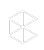The 2021 Clio Cloud Conference is Happening Now!

# Question:

• When a line item is chosen with no tax applied to it, but the other line items have the tax applied, the taxed amount seems to be calculated incorrectly.

# Environment:

• Clio Manage

• N/A

Tax is calculated on the total amount (time*rate) of the line items that have tax selected to be applied individually to them. That is, tax is not calculated based on the 'Sub-total' showing on the bill, but rather on the sub-total of the line items that have tax applied to them.

Eg:In the invoice above, the sub-total showing on the bill is 2070.

However, as highlighted in the image, tax is only applied to 5 of the line items (Excludes the \$12.50 line item. The taxable amount therefore, is \$2,057.50  [ie, 2070-12.50],and not \$ 2070.00

Seeing the sub-total of 2070 on the invoice (see below), and the tax-rate of 13% under it, might lead the reader to assume the taxed amount should then be (13% 2070 = \$269.1), which is not the case, as the taxable amount is \$2,057.50, and the taxed amount is therefore (13% 2057.50 = \$267.48)